## Example Questions

← Previous 1

### Example Question #1 : Factoring Equations

Solve 8x2 – 2x – 15 = 0

x = -3/2 or 5/4

x = 3/2 or -5/4

x = -3/2 or -5/4

x = 3/2 or 5/4

x = 3/2 or -5/4

Explanation:

The equation is in standard form, so a = 8, b = -2, and c = -15.  We are looking for two factors that multiply to ac or -120 and add to b or -2.  The two factors are -12 and 10.

So you get (2x -3)(4x +5) = 0.  Set each factor equal to zero and solve.

### Example Question #2 : Factoring Equations

If (x+ 2) / 2 = (x2 - 6x - 1) / 5, then what is the value of x?

4

3

2

-2

-3

-2

Explanation:

(x+ 2) / 2 = (x2 - 6x - 1) / 5. We first cross-multiply to get rid of the denominators on both sides.

5(x2 + 2) = 2(x2 - 6x - 1)

5x2 + 10 = 2x2 - 12x - 2 (Subtract 2x2, and add 12x and 2 to both sides.)

3x2 + 12x + 12 = 0 (Factor out 3 from the left side of the equation.)

3(x2 + 4x + 4) = 0 (Factor the equation, knowing that 2 + 2 = 4 and 2*2 = 4.)

3(x + 2)(x + 2) = 0

x + 2 = 0

x = -2

### Example Question #3 : Factoring Equations

Which of the following is a factor of the polynomial x2 – 6x + 5?

x + 2

x – 8

x + 1

x – 6

x – 5

x – 5

Explanation:

Factor the polynomial by choosing values that when FOIL'ed will add to equal the middle coefficient, 3, and multiply to equal the constant, 1.

x2 – 6+ 5 = (x – 1)(x – 5)

Because only (x – 5) is one of the choices listed, we choose it.

### Example Question #4 : Factoring Equations

7 times a number is 30 less than that same number squared. What is one possible value of the number?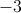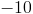Explanation: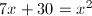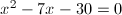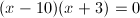Either: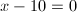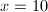or: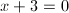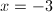### Example Question #5 : Factoring Equations

Which of the following is equivalent to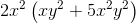?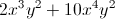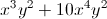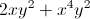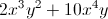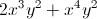Explanation:

The answer is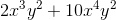.

To determine the answer,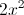must be distrbuted,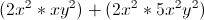. After multiplying the terms, the expression simplifies to.

### Example Question #1 : How To Factor An Equation

For what value of b is the equation b2 + 6b + 9 = 0 true?

3

3

5

0

3

Explanation:

### Example Question #7 : Factoring Equations

What is the solution to: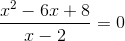1

2

6

4

0

4

Explanation:

First you want to factor the numerator from x– 6x + 8 to (x – 4)(x – 2)

Input the denominator (x – 4)(x – 2)/(x – 2) = (x – 4) = 0, so x = 4.

### Example Question #8 : Factoring Equations

What is the value ofwhere: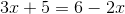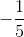Explanation:

The question asks us to find the value of, because it is in a closed equation, we can simply put all of the whole numbers on one side of the equation, and all of thecontaining numbers on the other side.

We utilize opposite operations to both sides by addingto each side of the equation and get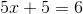Next, we subtractfrom both sides, yielding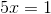Then we divide both sides byto get rid of thaton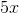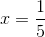### Example Question #9 : Factoring Equations

Factor the following equation: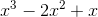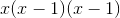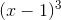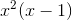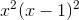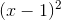Explanation:

First we factor out an x then we can factor the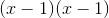### Example Question #19 : Simplifying Algebraic Expressions

Which of the following equations is NOT equivalent to the following equation?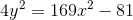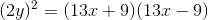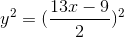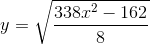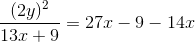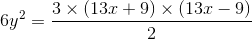Explanation:

The equation presented in the problem is:We know that: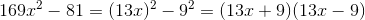Therefore we can see that the answer choiceis equivalent to.is equivalent to. You can see this by first combining like terms on the right side of the equation: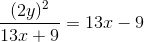Multiplying everything by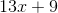, we get back to:We know from our previous work that this is equivalent to.is also equivalentsince both sides were just multiplied by. Dividing both sides by, we also get back to:.

We know from our previous work that this is equivalent to.is also equivalent tosince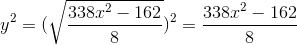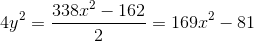Onlyis NOT equivalent tobecause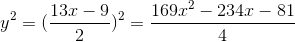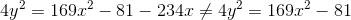← Previous 1